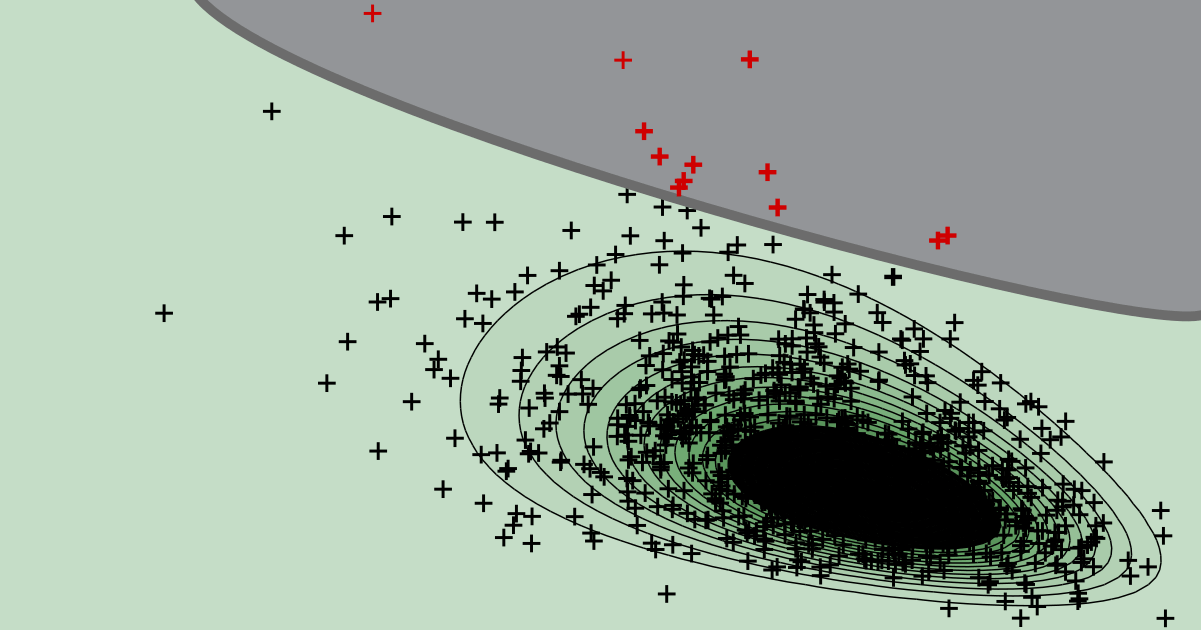# Monte Carlo simulation

Probabilistic modeling

## Does it make a difference whether or not a discretized Poisson process is used in Bayesian post-processing?

Even though the underlying posterior distributions are of different type, the results will be the same if the rate of the Poisson process is sufficiently small and weakly informative and consistent priors are used.

ONLINE TOOLReliability analysis

## Post-processing of Monte Carlo simulation

Use our web app to quantify the uncertainty about the probability of failure based on a conducted Monte Carlo simulation.
try it ...

Reliability analysis

## Why should you avoid Normal confidence intervals for Monte Carlo simulation?

The underlying distribution can be highly skewed, even if the total number of samples in the Monte Carlo simultion is very large. This is why the Normal approximation often performs poorly in practice.

Reliability analysis

## Why to avoid the coefficient of variation in the context of a Monte Carlo simulation?

The distribution quantifying the uncertainty about the probability of failure can be highly skewed, even for a large number of samples. The coefficient of variation is easier to interprete for symmetric distributions.

STRUREL

## How to employ Monte Carlo simulation in COMREL?

After a brief overview of Monte Carlo simulation (MCS) for structural reliability, we explain the details of conducting a MCS in COMREL.

Reliability analysis

## How to interpret the outcome of a Monte Carlo simulation if no failures occurred?

Even if Monte Carlo simulation returns not a single sample in the failure domain, we can still quantify the uncertainty about whether a specified target reliability level is maintained.

Reliability analysis

## How to assess the uncertainty in the outcome of a Monte Carlo simulation?

We explain a Bayesian post-processing step for MCS. It is ideally suited to quantify the uncertainty and to evaluate credible intervals for the probability of failure.

Reliability analysis

## How large is the statistical uncertainty in the estimate of a Monte Carlo simulation?

For a given probability of failure, the variance and coefficient of variation of the Monte Carlo estimate can be evaluated analytically. From this, the total number of samples required to maintain a target coefficient of variation can be deduced.

Reliability analysis

## Why is Monte Carlo simulation so robust?

Monte Carlo simulation is a very robust structural reliability method because its performance depends solely on the total number of samples and the underlying probability of failure.